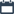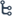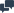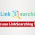Welcome to Instructbd

## Monday, 6 June 2016

•01:33:00
•••1 comment

## [Book] A First Course in Differential Equations with Modeling Applications By Dennis G. Zill

#### This book is mainly focus on Differential Equations and Modeling application. The authors of this book tried to write this book easily for better understand of the students. But Beginner level students have been facing some problems to solve this math because most of the section are formed with Partial Differential, First order Differential Equations, Second Order or Higher Order Differential Equations and Boundary Equations problems. So that If you have don't have any previous idea about differential method you are not able to understand the math of this book and if you don't able to solve these differential problem from these book , you can't learn the modeling application which are solved using differential method. Moreover,  In this book, Authors do not leave much space for explanations and examples, the explanations this book does provide are extremely difficult to follow, and the examples given are mostly skipping over a majority of the steps involved in solving the problems. For this reason, Beginners level students needs a guide or decent teacher to helps you solve this math. On top of everything, You must have interest in learning. If you want to learn something than you have to practicing the math more and more time. More practicing makes a student perfect.

Title: A First Course in Differential Equations with Modeling Applications
Author : Dennis G. Zill

Last Published: 2013

Edition: 10th

Total Pages: 489

Size : 12 MB

#### Content:

• Introduction To Differential Equations
• First-Order Differential Equations
• Modeling With First-Order Differential Equations
• High-Order Differential Equations
• Modeling with Higher-Order Differential Equations
• Series Solutions of Linear Equations
• The Laplace Transform
•  System of Linear First-Order Differential Equations
• Numerical Solutions of Ordinary Differential Equations
• Appendixes

(you can also read this book online)

#### 1 comment:

1.